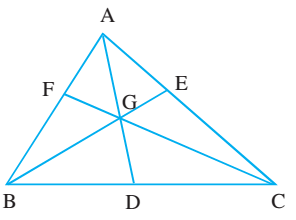# Medians of a Triangle

A line joining a vertex to the mid point of the opposite side of a triangle is called its median. Three medians can be drawn in a triangle.

Medians of a triangle pass through the same point, which divides each of the medians in the ratio 2:1. The point of concurrency G is called the centroid of the triangle.